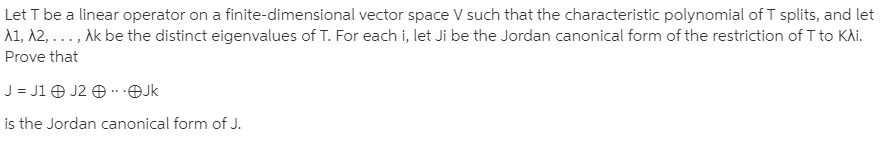# Let T be a linear operator on a finite-dimensional vector space V such that the characteristic polynomial of T splits, and letA1, A2, . .. , Ak be the distinct eigenvalues of T. For each i, let Ji be the Jordan canonical form of the restriction of T to KAi.Prove thatJ = J1 O J2 O -.OJkis the Jordan canonical form of J.

Question
1 viewshelp_outlineImage TranscriptioncloseLet T be a linear operator on a finite-dimensional vector space V such that the characteristic polynomial of T splits, and let A1, A2, . .. , Ak be the distinct eigenvalues of T. For each i, let Ji be the Jordan canonical form of the restriction of T to KAi. Prove that J = J1 O J2 O -.OJk is the Jordan canonical form of J. fullscreen

### This question hasn't been answered yet.